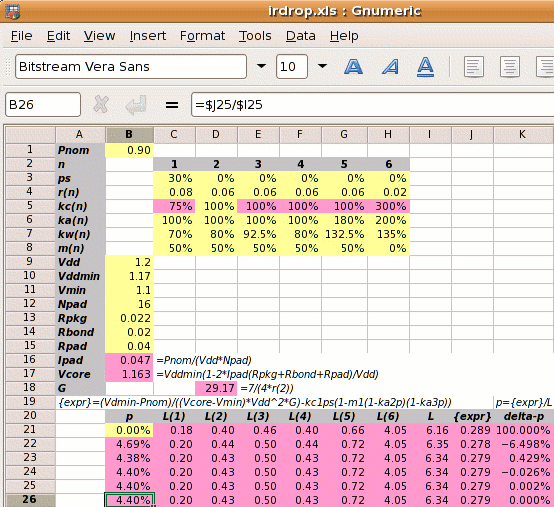Step 1: Calculate Ipad and Vcore:

0.9⁄(1.2×16) = 0.047A
Vcore =
1.17×(1−2×0.047×(0.022+0.02+0.04)⁄1.2
1.163V

Step 2: Calculate the reference power supply conductance G:

G =
 7 4×r2

7 ⁄ (4 × 0.06) =  29.17 mhos

Step 3 is to set out the values of kan, kwn, kcn and mn for each metal layer, and use these to calculate the value of L.

metal layer 1 2 3 4 5 6
kan 100% 100% 100% 100% 180% 200%
power metal allocated coefficient
kwn  70%  80% 92.5%  80% 132.5% 135%
power metal used coefficient
kcn  75%¹ 100% 100% 100% 100% 300%²
conductivity coefficient
mn  50%  50%  50%  50%  50%   0%
core area blocked
¹75%=.06/.08; ²300%=.06/.02

The value of L depends on p which we don't know. We iterate to the solution and use p=0 for the first estimate.

 L = kw1kc1(1-ps)(1-m1(1-ka2p)(1-ka3p))+ kw2kc2(1-m2(1-ka2p)(1-ka3p))+ kw3kc3(1-m3(1-ka2p)(1-ka3p))+ kw4kc4(1-m4(1-ka2p)(1-ka3p))+ kw5kc5(1-m5(1-ka2p)(1-ka3p))+ kw6kc6(1-m6(1-ka2p)(1-ka3p)) = ( 0.18 + 0.4 + 0.46 + 0.4 + 0.66 + 4.05 ) = 6.16

Step 4: Calculate the power strap allocation percentage p. The solution must be iterated, and the calculation below shows the first iteration.

 m1′ = m1×(1-ka2p)(1-ka3p)
p =
 { Vddmin×Pnom −kc1×ps(1−m1′) } × 1 (Vcore−Vmin)×Vdd2×G L
 { 1.17×0.9 −0.75×0.3×(1−0.5) } × 1 (1.161−1.1)×1.22×29.17 6.16
(0.401−0.112)×0.162 = 4.69%

As shown on the right, a spreadsheet can be used to iterate to the answer of p=4.40%.

The calculation of the die size including straps is on the next page.

Design Attribute Typical Value
Pnom core power consumption 0.9W
ps fraction of metal-1 in the standard cells used for power supplies 30% (for wsclib)
r1 resistivity of metal-1 in ohms per square 0.08Ω per sq.
r2-5 resistivity of metal-2 to metal-5 in ohms per square 0.06Ω per sq.
r6 resistivity of metal-6 in ohms per square 0.02Ω per sq.
ka1-4
 user defined   ratio of metal layer n allocated to power metal-2 allocated to power
100%
ka5 180%
ka6 200%
kw1
 user defined   ratio of metal layer n used for power metal-2 allocated to power
70%
kw2,4 80%
kw3 92.5%
kw5 132.5%
kw6 135%
m1-5 percentage of metal layers 1-5 which is blocked 50%
m6 percentage of metal layer 6 which is blocked 0%
Vdd the nominal supply voltage 1.2V
Vddmin minimum supply voltage, 2.5% less than nominal 1.17V
Vmin the desired voltage at the centre of the die 1.1V